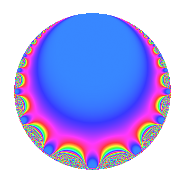# Properties

 Label 9.4.aLevel 9 Weight 4 Character orbit a Rep. character $$\chi_{9}(1,\cdot)$$ Character field $$\Q$$ Dimension 1 Newforms 1 Sturm bound 4 Trace bound 0

# Related objects

## Defining parameters

 Level: $$N$$ = $$9 = 3^{2}$$ Weight: $$k$$ = $$4$$ Character orbit: $$[\chi]$$ = 9.a (trivial) Character field: $$\Q$$ Newforms: $$1$$ Sturm bound: $$4$$ Trace bound: $$0$$

## Dimensions

The following table gives the dimensions of various subspaces of $$M_{4}(\Gamma_0(9))$$.

Total New Old
Modular forms 5 2 3
Cusp forms 1 1 0
Eisenstein series 4 1 3

The following table gives the dimensions of the cuspidal new subspaces with specified eigenvalues for the Atkin-Lehner operators and the Fricke involution.

$$3$$Dim.
$$+$$$$1$$

## Trace form

 $$q$$ $$\mathstrut -\mathstrut 8q^{4}$$ $$\mathstrut +\mathstrut 20q^{7}$$ $$\mathstrut +\mathstrut O(q^{10})$$ $$q$$ $$\mathstrut -\mathstrut 8q^{4}$$ $$\mathstrut +\mathstrut 20q^{7}$$ $$\mathstrut -\mathstrut 70q^{13}$$ $$\mathstrut +\mathstrut 64q^{16}$$ $$\mathstrut +\mathstrut 56q^{19}$$ $$\mathstrut -\mathstrut 125q^{25}$$ $$\mathstrut -\mathstrut 160q^{28}$$ $$\mathstrut +\mathstrut 308q^{31}$$ $$\mathstrut +\mathstrut 110q^{37}$$ $$\mathstrut -\mathstrut 520q^{43}$$ $$\mathstrut +\mathstrut 57q^{49}$$ $$\mathstrut +\mathstrut 560q^{52}$$ $$\mathstrut +\mathstrut 182q^{61}$$ $$\mathstrut -\mathstrut 512q^{64}$$ $$\mathstrut -\mathstrut 880q^{67}$$ $$\mathstrut +\mathstrut 1190q^{73}$$ $$\mathstrut -\mathstrut 448q^{76}$$ $$\mathstrut +\mathstrut 884q^{79}$$ $$\mathstrut -\mathstrut 1400q^{91}$$ $$\mathstrut -\mathstrut 1330q^{97}$$ $$\mathstrut +\mathstrut O(q^{100})$$

## Decomposition of $$S_{4}^{\mathrm{new}}(\Gamma_0(9))$$ into irreducible Hecke orbits

Label Dim. $$A$$ Field CM Traces A-L signs $q$-expansion
$$a_2$$ $$a_3$$ $$a_5$$ $$a_7$$ 3
9.4.a.a $$1$$ $$0.531$$ $$\Q$$ $$\Q(\sqrt{-3})$$ $$0$$ $$0$$ $$0$$ $$20$$ $$+$$ $$q-8q^{4}+20q^{7}-70q^{13}+2^{6}q^{16}+\cdots$$